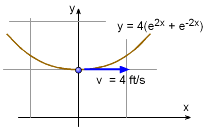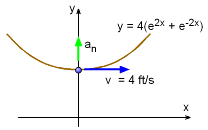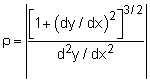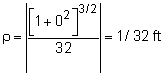Ch 1. Particle General Motion Multimedia Engineering Dynamics Position,Vel & Accel. Accel. varyw/ Time Accel. Constant Rect. Coordinates Norm/Tang. Coordinates Polar Coordinates RelativeMotion
 Chapter - Particle - 1. General Motion 2. Force & Accel. 3. Energy 4. Momentum - Rigid Body - 5. General Motion 6. Force & Accel. 7. Energy 8. Momentum 9. 3-D Motion 10. Vibrations Appendix Basic Math Units Basic Equations Sections Search eBooks Dynamics Fluids Math Mechanics Statics Thermodynamics Author(s): Kurt Gramoll ©Kurt GramollDYNAMICS - EXAMPLEParticle Motion along Path Example A particle moves along a wire that follows the curve y = 4(e2x + e-2x) at a constant velocity of 4 m/s. What is the normal acceleration of the particle when x = 0? SolutionPartical at x = 0 Since the velocity is constant, there is no acceleration tangent to its path. However, there is acceleration normal (perpendicular) to the path as given by,       an = v2/ρ Need to determine, ρdy/dx = d[ 4(e2x + e-2x) ] / dx               = 8 (e2x - e-2x )      d2y/dx2 = d[ 8(e2x - e-2x ) ] / dx                  = 16 (e2x + e-2x )      (dy/dx)x=0 = 8(1 - 1) = 0      (d2y/dx2)x=0 = 16(1 + 1) = 32Substituting back into the acceleration equation,      an = v2/ρ = 42 (32) an = 512 ft/s2

Practice Homework and Test problems now available in the 'Eng Dynamics' mobile app
Includes over 400 problems with complete detailed solutions.
Available now at the Google Play Store and Apple App Store.